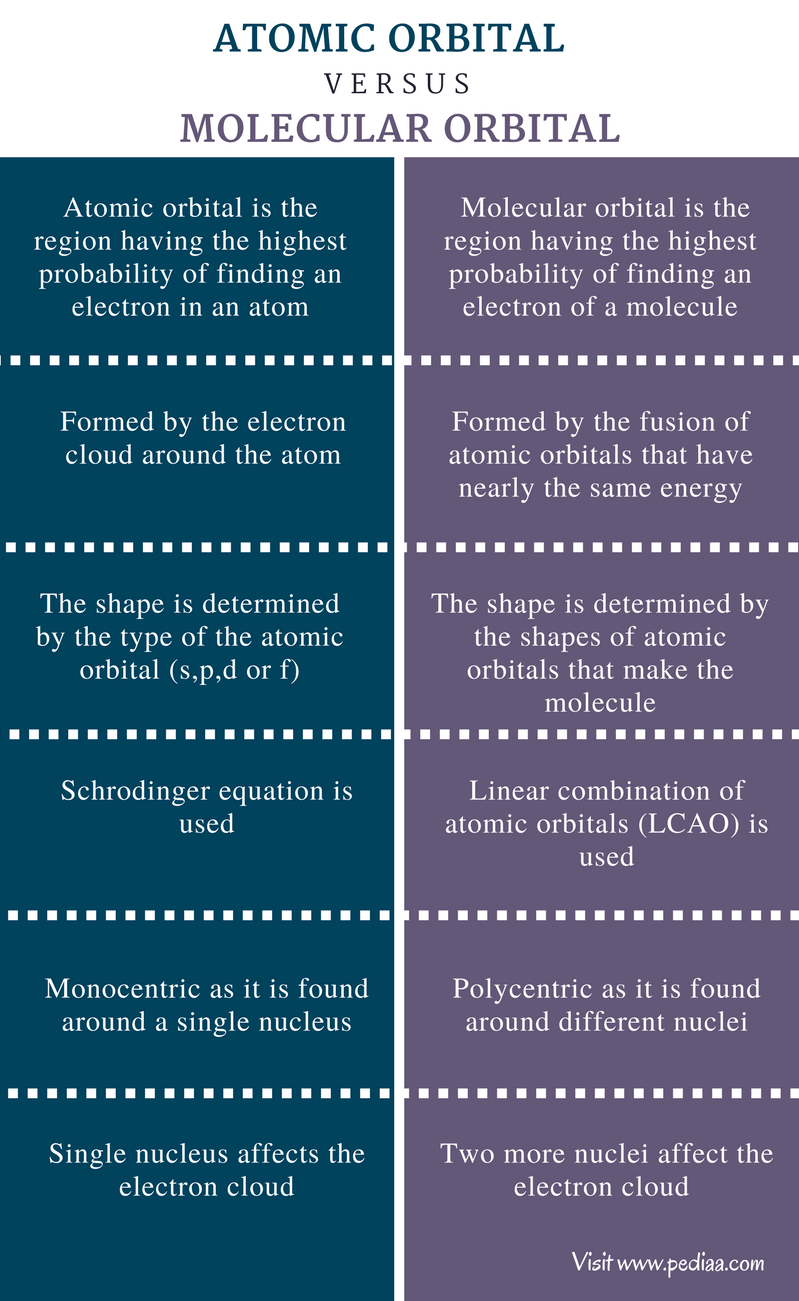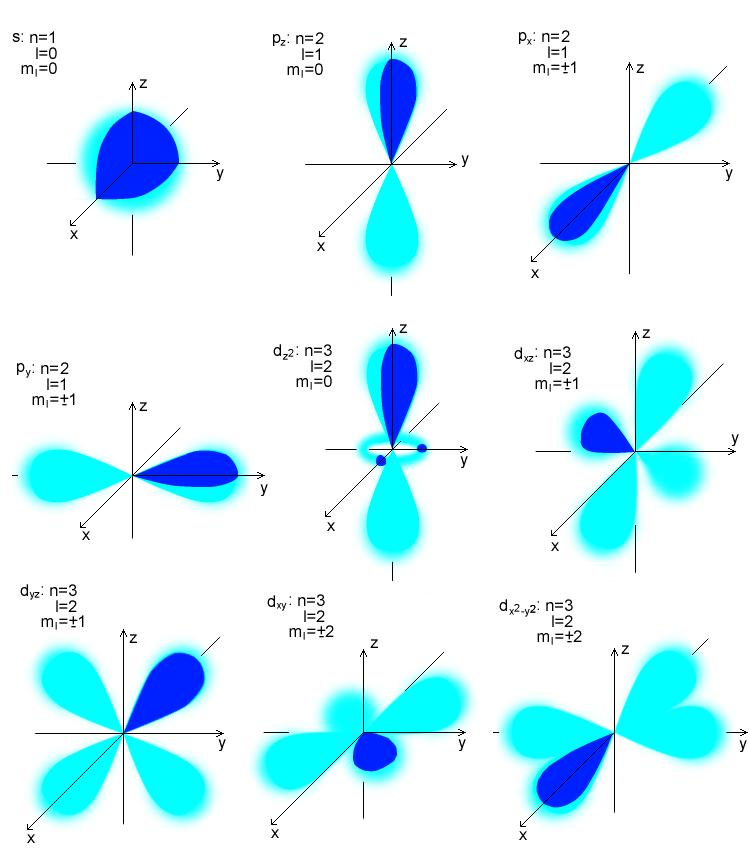# Difference Between Atomic Orbital and Molecular Orbital

## Main Difference – Atomic Orbital vs Molecular Orbital

Orbital is defined as a region where the probability of finding an electron is high. Atoms have their own electrons rotating around the nucleus. When these orbitals are overlapped to form molecules through the bonding, the orbitals are called molecular orbitals. Valence bond theory and molecular orbital theory explains the properties of atomic and molecular orbitals, respectively. Orbitals can hold a maximum of two electrons. The main difference between atomic and molecular orbital is that the electrons in an atomic orbital are influenced by one positive nucleus, while the electrons of a molecular orbital are influenced by the two or more nuclei depending upon the number of atoms in a molecule.

1. What is Atomic Orbital
– Definition, Characteristics, Properties
2. What is Molecular Orbital
– Definition, Characteristics, Features
3. What is the difference between Atomic Orbital and Molecular Orbital## What is an Atomic Orbital

The Atomic orbital is a region having the highest probability of finding an electron. Quantum mechanics explain the probability of the location of an electron of an atom. It does not explain the exact energy of an electron at a given prompt of time. It is explained in Heisenberg’s uncertainty principle. The electron density of an atom can be found from the solutions of the Schrodinger equation. An atomic orbital can have a maximum of two electrons. Atomic orbitals are labeled as s, p, d, and f sublevels. These orbitals have different shapes. The s orbital is spherical and hold a maximum of two electrons. It has one sub-energy level. The p orbital is dumbbell shaped and can hold up to six electrons. It has three sub energy levels. The d and f orbitals have more complex shapes. The d level has five sub-energy groups and holds up to 10 electrons, while f level has seven sub energy levels and can hold a maximum of ten and fifteen electrons. The energies of orbitals are in order of s<p<d<f.Figure 1: Atomic orbital types

## What is a Molecular Orbital

The properties of molecular orbitals are explained by the molecular orbital theory. It was first proposed by F. Hund and R.S. Mulliken in 1932. According to molecular orbital theory, when atoms are fused to form a molecule, the overlapping atomic orbitals lose their shape due to the effect of nuclei. The new orbitals present in the molecules are now called molecular orbitals. Molecular orbitals are formed by the combination of nearly the same energy atomic orbitals. Unlike atomic orbitals, the molecular orbitals do not belong to a single atom in a molecule but belong to nuclei of all the atoms which make the molecule. Thus, the nuclei of different atoms behave as a polycentric nucleus. The final shape of the molecular orbital depends on the shapes of atomic orbitals that make the molecule. According to Aufbau rule, the molecular orbitals are filled from low energy orbital to high energy orbital. Like an atomic orbital, a molecular orbital can hold a maximum number of two electrons. However, as per Pauli’s principle, these two electrons must have opposite spin. The behavior of the electron in a molecular orbital can be described by using the Schrodinger equation. However, due to the complexity of molecules, the application of Schrodinger equation is quite difficult. Hence, scientists have developed a method for approximate evaluation of the behavior of electrons in a molecule. The method is called linear combination of atomic orbitals (LCAO) method.Figure 2: Formation of molecular orbital

## Difference Between Atomic Orbital and Molecular Orbital

### Definition

Atomic Orbital: Atomic orbital is the region having the highest probability of finding an electron in an atom.

Molecular Orbital: Molecular orbital is the region having the highest probability of finding an electron of a molecule.

### Formation

Atomic Orbital: Atomic orbitals are formed by the electron cloud around the atom.

Molecular Orbital: Molecular orbitals are formed by the fusion of atomic orbitals that have nearly the same energy.

### Shape

Atomic Orbital: The shape of atomic orbitals is determined by the type of the atomic orbital (s,p,d or f).

Molecular Orbital: The shape of the molecular orbital is determined the shapes of atomic orbitals that make the molecule.

### Describing the Electron Density

Atomic Orbital: Schrodinger equation is used.

Molecular Orbital: Linear combination of atomic orbitals (LCAO) is used.

### Nucleus

Atomic Orbital: Atomic orbital is monocentric as it is found around a single nucleus.

Molecular Orbital: Molecular orbital is polycentric as it is found around different nuclei.

### Effect of Nucleus

Atomic Orbital: Single nucleus affects the electron cloud in atomic orbitals

Molecular Orbital: Two more nuclei affect the electron cloud in molecular orbitals.

### Summary

Both atomic and molecular orbitals are regions having the highest electron densities in atoms and molecules, respectively. The properties of atomic orbitals are determined by the single nucleus of atoms, whereas those of molecular orbitals are determined by the combination of atomic orbitals that form the molecule. This is the main difference between atomic orbital and molecular orbital.

References:
1.Verma, N. K., Khanna, S. K., & Kapila, B. (2010). Comprehensive chemistry XI. Laxmi publications.
2.Ucko, D. A. (2013). Basics for chemistry. Elsevier.
3.Mackin, M. (2012). Study Guide to Accompany Basics for Chemistry. Elsevier.

Image Courtesy:
1. “H atom orbitaly” By Pajs – Own work (Public Domain) via Commons Wikimedia
2. “Molecular orbitals sq” By Sponk (talk) – Own work (Public Domain) via Commons Wikimedia